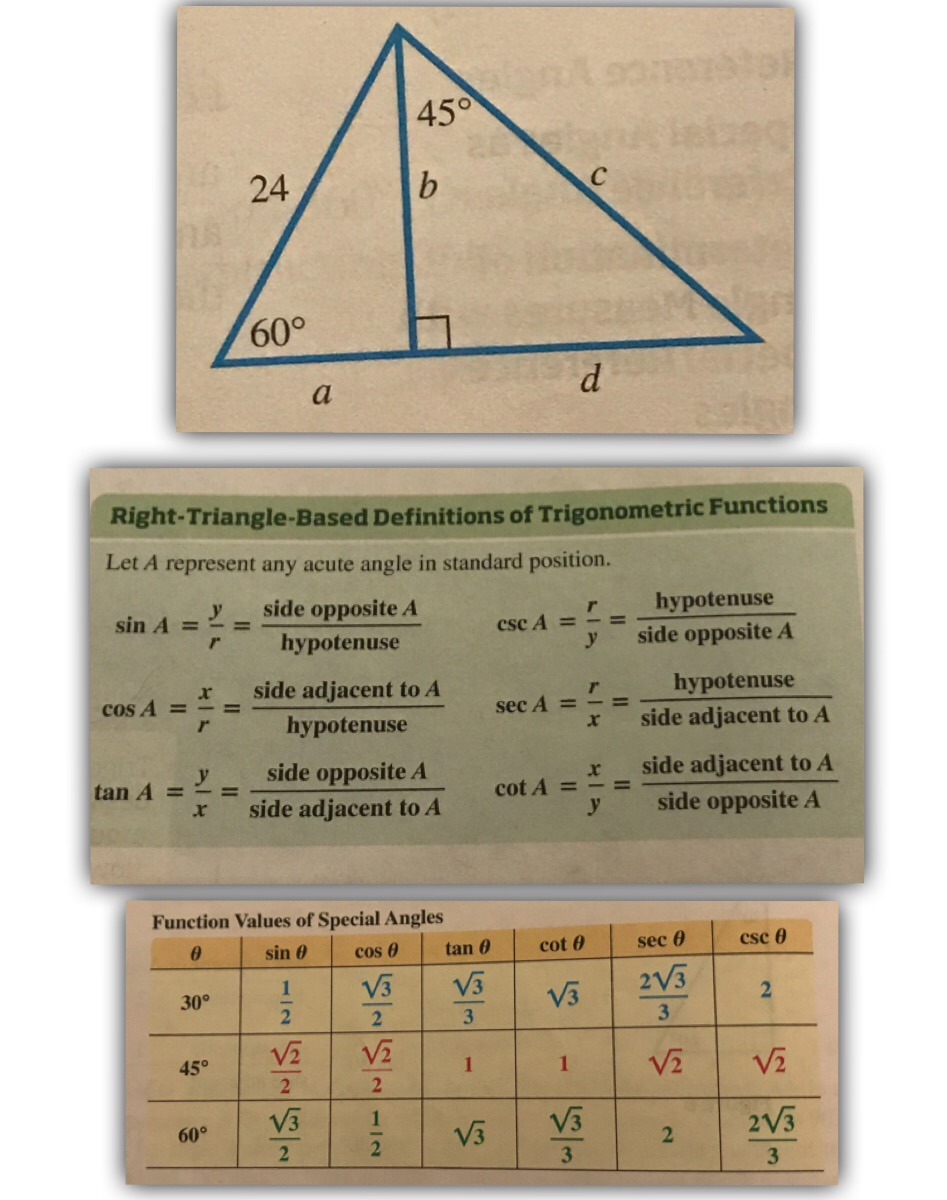# 45° 24 60° Right-Triangle-Based Definitions of Trigonometric Functions Let A represent any acute angle in standard position. sin A side opposite A hypotenuse se 4- -= r hypotenuse x side adjacent to A r hypotenuse y side opposite A hypotenuse COSA =-= sec A =-= x side adjacent to A tan A cot A = x = side adjacent to A x-sideadjacenttoA, y side opposite A Function Values of Special Angles sin θ | cos θ | tan θ | cot θ sec θ cse θ 30° 阪|1|阪 V3 60°

Question

Find the exact values for the unknown sides. You can find the sides in any order.

Use the green box and the yellow table to help.help_outlineImage Transcriptionclose45° 24 60° Right-Triangle-Based Definitions of Trigonometric Functions Let A represent any acute angle in standard position. sin A side opposite A hypotenuse se 4- -= r hypotenuse x side adjacent to A r hypotenuse y side opposite A hypotenuse COSA =-= sec A =-= x side adjacent to A tan A cot A = x = side adjacent to A x-sideadjacenttoA, y side opposite A Function Values of Special Angles sin θ | cos θ | tan θ | cot θ sec θ cse θ 30° 阪|1|阪 V3 60° fullscreen

### Want to see this answer and more?

Experts are waiting 24/7 to provide step-by-step solutions in as fast as 30 minutes!*